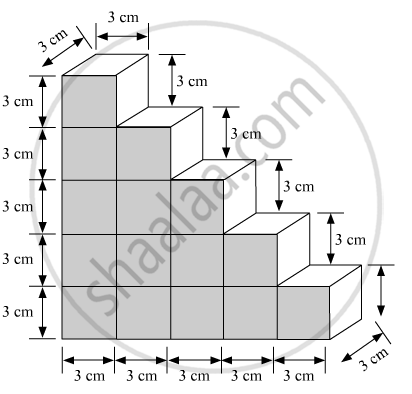# A Child Playing with Building Blocks, Which Are of the Shape of the Cubes, Has Built A Structure as Shown in Fig. - Mathematics

A child playing with building blocks, which are of the shape of the cubes, has built a structure as shown in Fig. 18.12 If the edge of each cube is 3 cm, find the volume of the structure built by the child.#### Solution 1

Volume of each cube = edge x edge x edge
=3xx3xx3cm^3=27cm^3

Number of cubes in the surface structure= 15
∴Volume of the structure = 27xx15cm^3

=405cm^3

#### Solution 2

We have,

Number of boxes (n) = 15In the above structure we need to find the total volume

Edge of each cube (a) = 3 cm

Volume of each cube(v) = a3

=33

= 27 cm

Hence, total volume of the structure,

V=n xx v

=15 xx27

=405  cm^3

The volume of the structure built by the child is.405 cm3

Concept: Volume of a Cuboid
Is there an error in this question or solution?

#### APPEARS IN

RD Sharma Mathematics for Class 9
Chapter 18 Surface Areas and Volume of a Cuboid and Cube
Exercise 18.2 | Q 17 | Page 30

Share